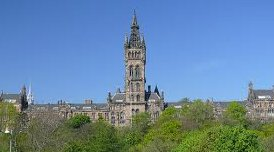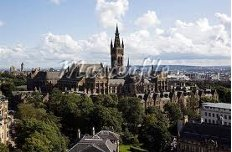Four different views of Glasgow University Tower

# Regular Two-graphs

The files

as their titles suggest, contain all regular two-graphs on at most 50 vertices that are known to me at this time. Here each two-graph is identified with its greatest descendant, where the ordering involved is the natural one obtained by expressing as binary integer the concatenation of the rows of the upper triangular part of the adjacency matrix. I also list the order of its automorphism group, and the orbits under the action of this group. In addition, I include the numbers of strongly regular graphs that are obtained either as descendants of the regular two-graph or, where appropriate, in its switching class. The strongly regular graphs are identified by their parameters which comprise the number of vertices and the eigenvalues of their Seidel Spectrum. This is simply the spectrum of its (-,+) adjacency matrix. The notation [x,y] means that there are x graphs with automorphism group of order y.

Perhaps the updated files concerning regular two-graphs on 36 and 50 vertices need some explanation. It is now known that there are precisely 227 regular two-graphs on 36 vertices ,  and the additional work  increased the known number of regular two-graphs on 50 vertices to 54 (6 self-complementary and 24 complementary pairs).

 E. Spence, Regular two-graphs on 36 vertices, Lin. Alg. Appl. 226-228 (1995), 459-497.

 Brendan McKay & E Spence, The Classification of Regular Two-graphs on 36 and 38 vertices, Australas. J. Combin.. 24 (2001), 293-300.

 E. Spence, Unpublished computer result, 1995